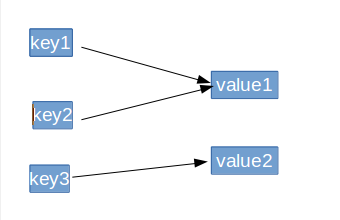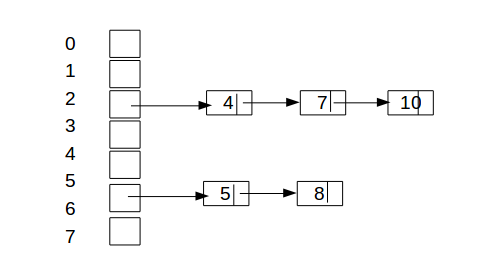26 篇文章 0 订阅

# 散列表分析（Java实现）

## 一、 散列表的原理## 三、解决“碰撞”的方法

### 1.开放地址法

key哈希后，发现该地值已被占用，可对该地址不断加1，直到遇到一个空的地址。

### 3.链地址法## 四、Java以链地址法实现散列表及分析

### 注意事项

• 扩容因子
散列表的地址使用率越大，发生“碰撞”的可能性就越大，一般在散列表地址使用率达到一定值的时候，就对散列表进行扩容，这个值称为扩容因子，为小数。

• 关键字key
关键字可以不为整形，但一般高级语言会把所有类型的key都转换为整形，而Java中有hashCode()方法，可以得到任何类型量的哈希值。



import java.util.Objects;

/**
* Created by max on 17-5-3.
*/
public class MyHashTable {

private static final int DEFAULT_INITAL_CAPACITY = 5;//定义的是默认长度

private static final float LOAD_FACTOR = 0.75f;//扩容因子

private Entry[] table = new Entry[DEFAULT_INITAL_CAPACITY];//初始化
private int size =0;//哈系表大小
private int use =0;//使用的地址数量

private class Entry{
int key;//关键字
int value;
Entry next;//链表

public Entry(int key,int value ,Entry entry)//构造函数
{
super();
this.key = key;
this.value = value;
this.next = entry;
}
}

public void put(int key,int value){//压入内容
int index =hash(key);//通过hash方法转换，采用的是直接法

if (table[index]==null)//说明位置未被使用
{
table[index] = new Entry(-1,-1,null);
}

Entry tmp = table[index];
if (tmp.next == null)//说明位置未被使用
{
table[index].next = new Entry(key,value,null);
size++;
use++;
{
resize();//扩容方法
}
}else{//已被使用，则直接扩展链表
for (tmp = tmp.next;tmp!=null;tmp = tmp.next)
{
int k =tmp.key;
if(k==key)
{
tmp.value = value;
return;
}
}

Entry temp = table[index].next;
Entry newEntry = new Entry(key,value,temp);
table[index].next = newEntry;
size++;
}

}
public void remove(int key) //删除，链表的中间值删除方法
{
int index =hash(key);
Entry e = table[index];
Entry pre = table[index];
if (e!=null&& e.next!=null)
{
for (e=e.next;e!=null;pre =e,e =e.next)
{
int k =e.key;
if(k==key)
{
pre.next = e.next;
size--;
return;
}
}
}
}

public int get(int key)//通过key提取value
{
int index = hash(key);
Entry e =table[index];
if (e!=null&&e.next!=null)
{
for (e=e.next;e!=null;e=e.next)
{
int k = e.key;
if (k ==key)
{
return e.value;
}
}
}
return -1;
}

public int size(){//返回元素个数
return size;

}

public int getLength(){//哈系表大小
return table.length;
}

private void resize() {
int newLength = table.length*2;
Entry[] oldTable = table;
table = new Entry[newLength];
use = 0;
for(int i =0 ;i<oldTable.length;i++)
{
if (oldTable[i]!=null&&oldTable[i].next !=null)
{
Entry e = oldTable[i];
while(null!=e.next)
{
Entry next = e.next;
int index =hash(next.key);
if (table[index]==null)
{
use++;
table[index] = new Entry(-1,-1,null);
}
Entry temp = table[index].next;
Entry newEntry =new Entry(next.key,next.value,temp);
table[index].next = newEntry;

e = next;
}
}
}

}

private int hash(int key) {//哈希方法
return key%table.length;
}

}


08-2510-262730
10-131万+
04-2252
11-034042
12-28228
04-1597
02-281329
05-062859
06-1783
10-31225
10-097555
12-023212
02-0499
08-2097
07-103万+
03-15337
05-10406点击重新获取扫码支付余额充值